# Iterative Approach for Creating Labeled Signal Sets with Reduced Human Effort

This example presents an iterative deep learning-based workflow to label signals with reduced human labeling effort.

Labeling signal data is a tedious and expensive task that requires much human effort. Finding ways to reduce this effort can significantly speed up the development of deep learning solutions for signal processing problems.

Consider the task of labeling regions of interest in a signal data set. A first approach consists of labeling the data by hand. This approach requires much time and effort. An alternative approach, explored in this example, treats the labeling process iteratively. At each iteration, a subset of signals is selected from the unlabeled data set and is sent to a pretrained deep network for automated labeling. A human labeler examines the resulting labels and corrects wrong labels. The validated labeled signals are added to a training data set to retrain the deep network with the extended training data.

At each iteration, the human labeler still has to visit and examine all the signals labeled by the network. However, the task changes from labeling signals from scratch to correcting inaccurate labels generated by a reliable network. This latter task requires considerably less human labeling effort. At each new iteration, the network is trained with more and more data, causing the prediction and labeling performance of the network to improve. Hence, at each iteration, less and less human intervention is required to correct labels.

### Data

This example considers the labeling of ECG signal regions using data publicly available in the QT Database  . The data consists of roughly 15 minutes of ECG recordings from a total of 105 patients. To obtain each recording, the examiners placed two electrodes on different locations on a patient's chest, resulting in a two-channel signal. The database provides signal region labels generated by an automated expert system . The labels correspond to the locations of P wave, T wave, and QRS complex regions in the ECG measurements. This example follows the procedure presented in Waveform Segmentation Using Deep Learning to train a bidirectional long short-term memory (BiLSTM) network that can classify ECG signal samples as belonging to one of the three regions of interest.

Download the data from the GitHub Repository. This example assumes that you have put the file in your temporary directory, whose location is specified by MATLAB®'s `tempdir` command. Unzip the datafile and load the data.

```unzip(fullfile(tempdir,'QT_Database-master.zip'),tempdir) load(fullfile(tempdir,'QT_Database-master','QTData.mat'))```

Use the `displayWaveformLabels` helper function to plot the first 1000 samples of the ECG signal from the first patient, with the regions labeled by the expert system overlaid in different colors. The dataset contains an extra label, 'N/A', that is used to identify signal samples that do not belong to any of the three regions of interest.

```patientID = 1; signalVals = getSignal(QTData,patientID); labelVals = getLabelValues(QTData,patientID,'WaveformLabels_Chan1'); displayWaveformLabels(signalVals(1,1:1000),labelVals.Value(1:1000)) ```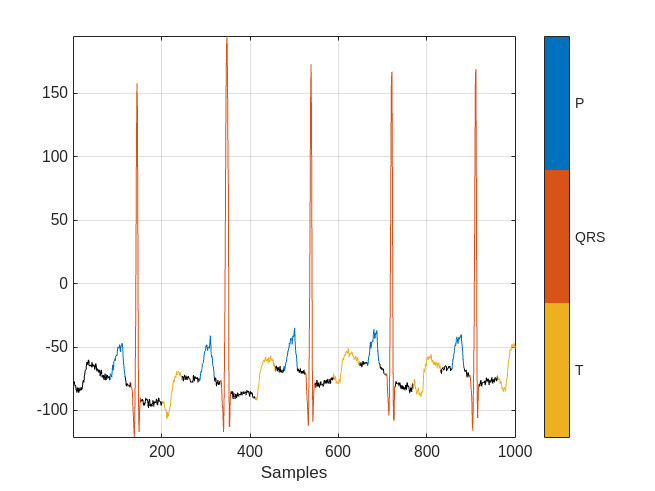Use the `resizeSignals` helper function to break the ECG signals and label vectors into frames, each of them 5000 samples long. The function then shuffles the frames and divides them into a training data set containing 70% of the data and a test data set containing 30% of the data.

```rng default [testFrames,testLabels,ecgFrames,ecgLabels,testInfo,ecgInfo] = resizeSignals(QTData,0.3);```

`ecgFrames` contains 6543 frames and `testFrames` contains 2768 frames. The variable `ecgInfo` stores the patient ID, the channel ID, and the sample index of each frame of the training data set. `testInfo` stores the same information for the test data set.

Transform the ECG frames to the time-frequency domain. Use the `featureExtraction` helper function to compute the Fourier synchrosqueezed transform of the ECG signal frames over the frequency range of interest, [0.5, 40] Hz, and standardize the resulting transforms by subtracting the mean and dividing by the standard deviation.

```Fs = QTData.SampleRate; testFrames = featureExtraction(testFrames,Fs); ecgFrames = featureExtraction(ecgFrames,Fs);```

This example shows that it is possible to decrease the human effort involved in signal labeling by iteratively training a deep network. At each iteration:

1. The network labels a subset of unlabeled data frames using previously labeled frames.

2. A human labeler corrects any labeling errors by hand.

3. The corrected labeling is added to the previously labeled frames.

4. The expanded set of labeled signals is used to train the network for the next iteration.

To make quantitative comparisons, simulate two scenarios:

• For the baseline scenario, in which a human being labels the entire dataset from scratch, train the network using the full labeled `ecgFrames` set.

• For the second scenario, pretend that the `ecgFrames` data is unlabeled and label it using the iterative method.

### Prediction Performance Using Fully Labeled ECG Data Set

Build a BiLSTM network and train it with the full labeled `ecgFrames` set to get a prediction-performance upper bound. As mentioned above, this approach requires brute-force labeling of the whole data set and hence the largest human labeling effort. Train the network with the labeled `ecgFrames` set and calculate the prediction accuracy on the test data set.

#### Network Architecture

Create a BiLSTM network using deep learning layers.

• Specify a `sequenceInputLayer` with a size as the number of features in the FSST of the signals, which is the total number of frequency-domain samples (40 in this example).

• Specify a `bilstmLayer` with 200 hidden nodes and set the `OutputMode` to `sequence` since each signal sample has a label.

• Specify a `fullyConnectedLayer` with an output size of 4 corresponding to the four categories, P wave, QRS complex, T wave, and N/A.

• Add a `softmaxLayer` and a `classificationLayer` to output the estimated labels.

```% Training with the full emulated unlabeled data set layers = [ ... sequenceInputLayer(size(ecgFrames{1},1)) bilstmLayer(200,'OutputMode','sequence') fullyConnectedLayer(4) softmaxLayer classificationLayer];```

Use `traningOptions` to specify the optimization solver and the hyperparameters to train the network. This example uses the ADAM optimizer and a mini-batch size of 50. Train the network using either a CPU or GPU. Using a GPU requires Parallel Computing Toolbox™ and a CUDA® enabled NVIDIA® GPU with compute capability 3.0 or higher. For information on other parameters, see `trainingOptions` (Deep Learning Toolbox). This example uses a GPU for training using the '`ExecutionEnvironment`' name-value pair.

```options = trainingOptions('adam', ... 'MaxEpochs',10, ... 'MiniBatchSize',50, ... 'ExecutionEnvironment','gpu', ... 'InitialLearnRate',0.01, ... 'LearnRateDropPeriod',6, ... 'LearnRateSchedule','piecewise', ... 'GradientThreshold',1, ... 'Shuffle','every-epoch',... 'Plots','training-progress',... 'Verbose',0);```

Train the network with the fully labeled `ecgFrames` data set.

`baselineNet = trainNetwork(ecgFrames,ecgLabels,layers,options);`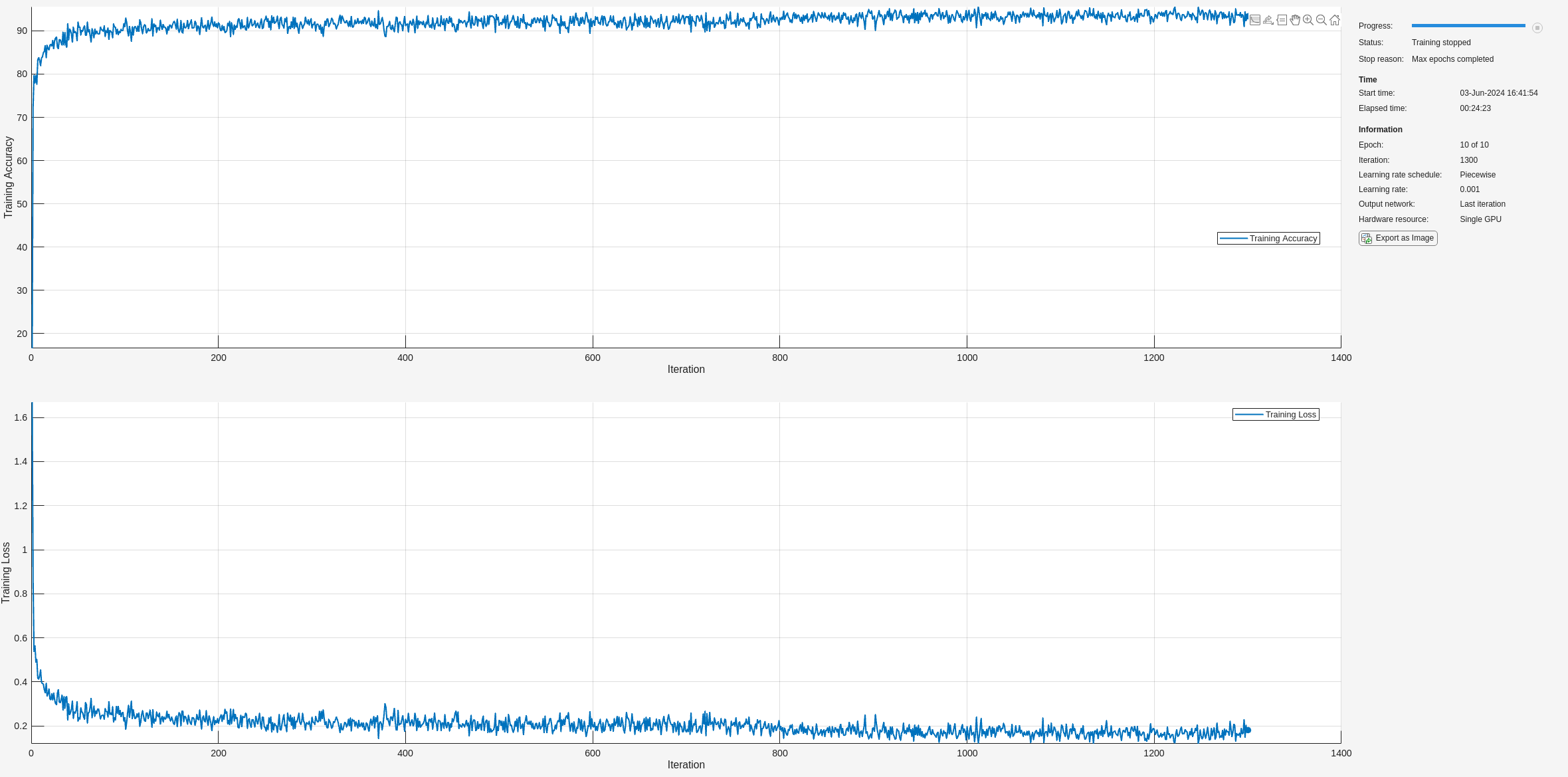Classify the test frames using the trained network and compute the mean prediction accuracy. The baseline prediction accuracy is about 95%.

```predictLabelsAll = classify(baselineNet,testFrames,'MiniBatchSize',50); accuracyAll = mean(cellfun(@(x,y)mean(x==y),predictLabelsAll,testLabels)); fprintf('The baseline prediction accuracy is %2.1f%%.\n',accuracyAll*100);```
```The baseline prediction accuracy is 94.2%. ```

### Iterative Labeling with Human in the Loop

To reduce the labeling effort, try an iterative approach: Pretend that the `ecgFrames` data set is initially unlabeled and that the data are labeled manually. In reality, the example uses the ground truth labels provided by the data set.

### Train an Initial Network

Start by selecting 25 frames from the `ecgFrames` set and labeling them manually. Train a BiLSTM network with this initial labeled set to serve as the initial step of the iterative process.

```numInitFrames = 25; currentTrainingSet = ecgFrames(1:numInitFrames,1); currentTrainingLabels = ecgLabels(1:numInitFrames);```

Set the training options to have more training epochs and a smaller mini-batch size because there are only 25 frames within the initial training data set.

```options = trainingOptions('adam', ... 'MaxEpochs',20, ... 'MiniBatchSize',5, ... 'ExecutionEnvironment','gpu', ... 'InitialLearnRate',0.01, ... 'LearnRateDropPeriod',6, ... 'LearnRateSchedule','piecewise', ... 'GradientThreshold',1, ... 'Shuffle','every-epoch', ... 'Plots','none',... 'Verbose',0);```

Train the BiLSTM network with the initial training data set and predict labels using the same test data set used to establish the performance baseline. The prediction accuracy of the networks is less than about 80%.

```initNet = trainNetwork(currentTrainingSet,currentTrainingLabels,layers,options); initPrediction = classify(initNet,testFrames,'MiniBatchSize',50); initAccuracy = mean(cellfun(@(x,y)mean(x==y),initPrediction,testLabels)); fprintf('The prediction accuracy is %2.1f%%.\n',initAccuracy*100);```
```The prediction accuracy is 78.3%. ```

#### Labeling

In the next step, select 200 new data frames from the `ecgFrames` set and feed them to the pretrained network, `initNet`, to label the signals automatically.

```iteration = 1; % Number of frames to label at each iteration numFrames = 200; % Select the next set of frames to label indexNext = numInitFrames+1:numInitFrames+numFrames; currentPrediction = classify(initNet,ecgFrames(indexNext),'MiniBatchSize',50);```

Evaluate the labeling results generated by the network and compare them to the ground truth. Use the third of the selected 200-sample data frames as example: Plot its first 750 samples overlaid with the ground-truth labels and the labels predicted by the network.

```idx = 3; info = ecgInfo{indexNext(idx)}; signal = QTData.Source{info.patientID}(info.channelID,info.indexID); groundTruthLabels = ecgLabels{indexNext(idx)}; predictedLabels = currentPrediction{idx}; subplot(2,1,1) displayWaveformLabels(signal,groundTruthLabels) xlim([1 750]) title('Ground Truth') subplot(2,1,2) displayWaveformLabels(signal,predictedLabels) xlim([1 750]) title('Labeling by Network')```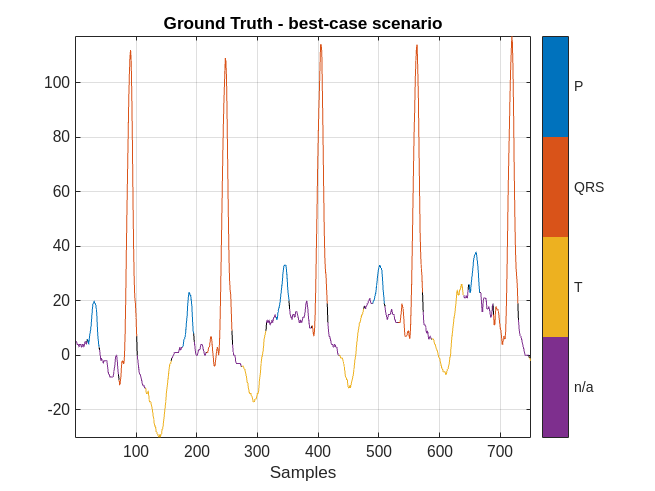The network did a good job labeling this frame. As a result, a human inspecting the results of the network can correct the predicted labels with little effort.

However, there are cases when the labeling performance of the network is not as strong. Consider the results obtained with the sixth of the selected 200-sample data frames.

```idx = 6; info = ecgInfo{indexNext(idx)}; signal = QTData.Source{info.patientID}(info.channelID,info.indexID); groundTruthLabels = ecgLabels{indexNext(idx)}; predictedLabels = currentPrediction{idx}; figure subplot(2,1,1) displayWaveformLabels(signal,groundTruthLabels) xlim([1 750]) title('Ground Truth') subplot(2,1,2) displayWaveformLabels(signal,predictedLabels) xlim([1 750]) title('Labeling by Network')```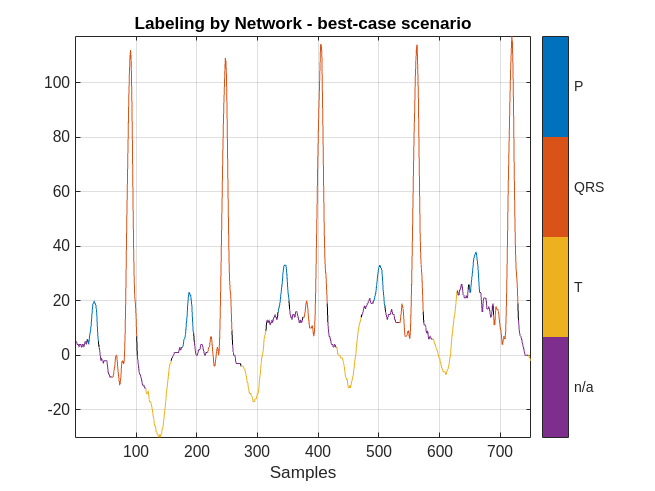The performance of the network on this signal is not as good. To inspect the results, a human labeler has to make several corrections to the predicted labels.

To quantify the correction effort for the 200 data frames, calculate the labeling error rate of the network and the average number of samples per frame that must be corrected by the human labeler.

```numSamplesPerFrame = 5000; networkLabelingErrorRate(iteration) = 1-mean(cellfun(@(x,y)mean(x==y),currentPrediction,ecgLabels(indexNext))); averageNumOfCorrectionsPerFrame(iteration) = networkLabelingErrorRate(iteration) * numSamplesPerFrame; fprintf('The average number of corrections per frame is %2.1f.\n',averageNumOfCorrectionsPerFrame(iteration));```
```The average number of corrections per frame is 1077.4. ```

For the first iteration, there are on average about 1000 samples per frame that must be corrected by a human.

At the end of the first iteration, the human will inspect the 200 frames and modify any label with incorrect values. With the help of the network and the human labeler, the data frames have correct labels at the end of the iteration.

On the next iteration, the 200 newly labeled frames can be added to the `currentTrainingSet` set to retrain the network and repeat the labeling iteration. This chart illustrates the workflow in each iteration after the first iteration: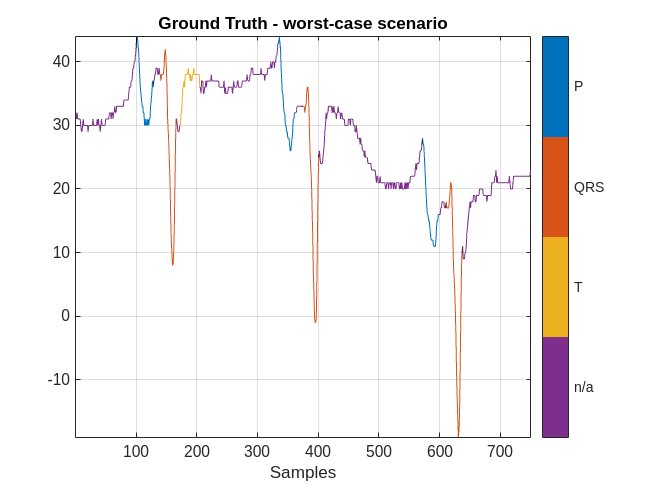#### Repeat Labeling Iterations

Extend the training set by adding the newly corrected labeled frames, select another 200 data frames to be labeled, and repeat the labeling iteration until the performance is satisfactory.

```% Include the initial training set and the 200 newly labeled data frames maxIter = 15; indexTraining = 1:numInitFrames+numFrames; networkAccuracy = zeros(1,15); networkAccuracy(iteration) = initAccuracy; options = trainingOptions('adam', ... 'MaxEpochs',20, ... 'MiniBatchSize',50, ... 'ExecutionEnvironment','gpu', ... 'InitialLearnRate',0.01, ... 'LearnRateDropPeriod',6, ... 'LearnRateSchedule','piecewise', ... 'GradientThreshold',1, ... 'Shuffle','every-epoch', ... 'Plots','none', ... 'Verbose',0); for iteration = 2:maxIter % Extended training data set currentTrainingSet = ecgFrames(indexTraining,1); % Emulate human correction by assigning ground-truth labels to the % extended training set currentTrainingLabels = ecgLabels(indexTraining); % Train network with extended training set currentNet = trainNetwork(currentTrainingSet,currentTrainingLabels,layers,options); % Predict labels for the test data set and calculate the accuracy to % compare to baseline performance currentTestSetPrediction = classify(currentNet,testFrames,'MiniBatchSize',50); networkAccuracy(iteration) = mean(cellfun(@(x,y)mean(x==y),currentTestSetPrediction,testLabels)); % Get another numFrames data frames for human labeler indexNext = indexTraining(end)+1:indexTraining(end)+numFrames; % Measure average number of human corrections per frame in this iteration currentPrediction = classify(currentNet,ecgFrames(indexNext),'MiniBatchSize',50); networkLabelingErrorRate(iteration) = 1-mean(cellfun(@(x,y)mean(x==y),currentPrediction,ecgLabels(indexNext))); averageNumOfCorrectionsPerFrame(iteration) = networkLabelingErrorRate(iteration) * numSamplesPerFrame; indexTraining = 1:indexNext(end); end```

### Labeling Performance

After 15 labeling iterations, there are 2825 data frames in `currentTrainingSet`, corresponding to about half of the 6543 data frames contained in the full `ecgDataset` set. The prediction accuracy of the network trained with 2825 frames is only about 1% lower than the baseline accuracy.

```accuDiff = accuracyAll-networkAccuracy(end); fprintf('The accuracy difference is %2.1f%%.\n',accuDiff*100);```
```The accuracy difference is 0.8%. ```

Plot the network prediction accuracy for the test data set with respect to the size of the training data set at each iteration. Show the upper bound of the accuracy obtained with the fully labeled data set. As more data frames are validated, the prediction accuracy of the network improves.

```figure examinedDataSize = 25:200:2825; plot(examinedDataSize,networkAccuracy,'*-') hold on % Prediction accuracy upper bound plot(examinedDataSize,ones(1,15)*accuracyAll,'r--') grid on xlabel('Training set size') title('Accuracy for the test data set') xlim([25 2825]) legend('Labeling Network','Upper Bound','Location','southeast')```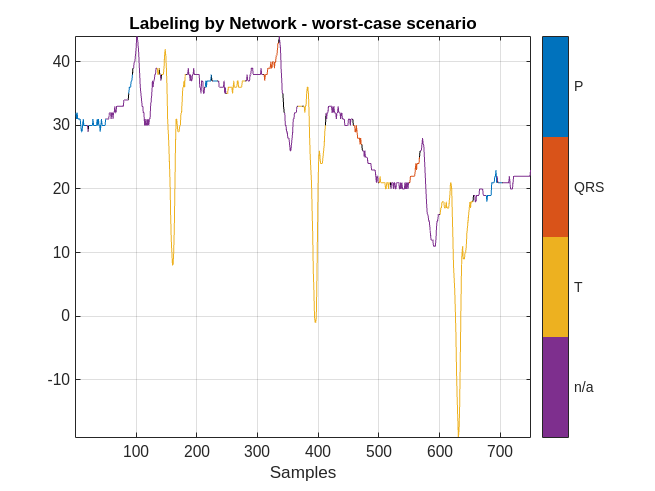At the end of each iteration, the average number of human corrections per frame decreases as the size of the training dataset increases. As more data frames are validated and used to train the network, less human effort is required to correct the labels of the selected frames. At the last iteration, there is an average of 300 samples per frame that require human correction.

```figure plot(examinedDataSize,averageNumOfCorrectionsPerFrame,'*-') grid on xlabel('Training set size') title('Average number of human corrections per frame') xlim([25 2825])```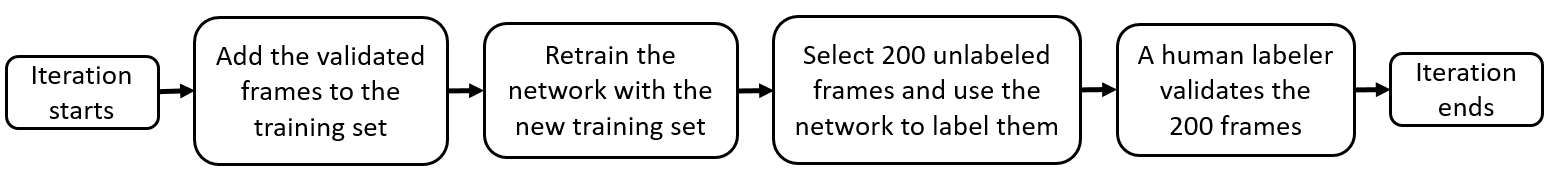Throughout all 15 labeling iterations, an average of about 500 signal samples per frame required human corrections.

`fprintf('The average number of corrections per frame is %2.1f.\n',mean(averageNumOfCorrectionsPerFrame));`
```The average number of corrections per frame is 465.7. ```

### Conclusion

This example showed that labeling only half of an ECG data set allows a deep network to achieve a prediction accuracy similar to that achieved by the same network when trained with the fully labeled data set. With the proposed iterative labeling workflow, a human labeler needs to look at only half of the data set and correct an average of 500 signal samples per frame. On the other hand, brute-force labeling requires looking at every frame in the data set and labeling all of its samples from scratch.

### References

 Goldberger, Ary L., Luis A. N. Amaral, Leon Glass, Jeffery M. Hausdorff, Plamen Ch. Ivanov, Roger G. Mark, Joseph E. Mietus, George B. Moody, Chung-Kang Peng, and H. Eugene Stanley. "PhysioBank, PhysioToolkit, and PhysioNet: Components of a New Research Resource for Complex Physiologic Signals." Circulation. Vol. 101, Number 23, 2000, pp. e215–e220. [Circulation Electronic Pages; http://circ.ahajournals.org/content/101/23/e215.full].

 Laguna, Pablo, Roger G. Mark, Ary L. Goldberger, and George B. Moody. "A Database for Evaluation of Algorithms for Measurement of QT and Other Waveform Intervals in the ECG." Computers in Cardiology. Vol.24, 1997, pp. 673–676.

 Laguna, Pablo, Raimon Jané, and Pere Caminal. "Automatic detection of wave boundaries in multilead ECG signals: Validation with the CSE database." Computers and Biomedical Research. Vol. 27, Number 1, 1994, pp. 45–60.